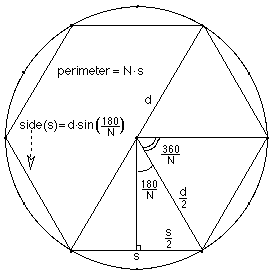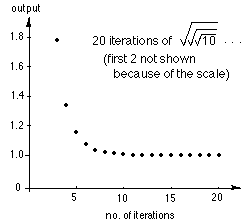## Answers for Chapter 10 Sample Problems

1. Kohler follows Archimedes
Kohler got the ratios p/d of 2.6, 3, and 3.1 for the 3-, 6- and 12-sided inscribed regular polygons. To generalize this ratio, let N = the # of sides of the polygon and since we are doubling the # of sides N = 3*2f, d= the diameter of the circle, and s = the length of one side of the polygon.From the diagram at the right, sin(180/N) = s/2/d/2 and s = d*sin(180/N) or in radians, s = d*sin(Pi/N). A program in Basic to get the first 10 ratios would be:
10 f = 0
20 N = 3*2f
30 Print N*sin(Pi/N)
40 f = f + 1
50 IF f>10 THEN STOP
60 GOTO 20
Running the program we get the infinite sequence 2.59807, 3, 3.1058, 3.1326, 3.1393, ... and after 10 loops, we get 3.14159... . Notice the first 3 are very close to what Kohler had. And notice that we are correct to 5 places for Pi!

4. Iterating the square root of a number.
This is the graph of iterating the squart root of 10 (at the right).A program in Basic, to get 19 iterations,
would be:

• 10 INPUT N
• 20 For c = 1 to 20
• 30 N = Sqrt(N)
• 40 PRINT N
• 50 Next c

6. Finding the square root of N by the iteration of the averaging method.
Gayla, a 6th grader wrote this program to get the square root of N , starting with G

• 10 C=0
• 20 INPUT N
• 30 INPUT G
• 40 G=(G+N/G)/2
• 50 C=C+1
• 60 PRINT G
• 70 If C is less than 19 THEN GOTO 40
• 80 END

Back to Ch. 10 problems
To order Don's materials
To choose sample problems from other chapters
Mathman home